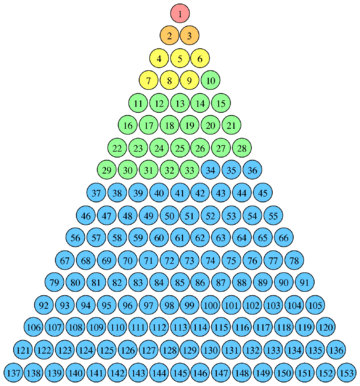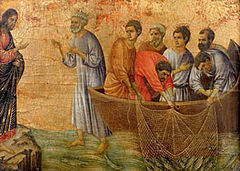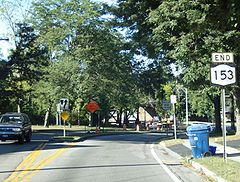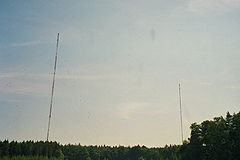# 153 (number)

﻿
153 (number)
153
Ordinal 153
Cardinal 153rd
Factorization$153 = 3^2 \cdot 17$
Divisors 1, 3, 9, 17, 51, 153
Roman numeral CLIII
Binary 10011001
Octal 231

One hundred (and) fifty-three is the natural number following one hundred (and) fifty-two and preceding one hundred (and) fifty-four.

## Mathematical propertiesThe number 153 is the 17th triangular number. The colours show that 153 is also the sum of the first five positive factorials.

As a triangular number, 153 is the sum of the first 17 integers, and it also the sum of the first five positive factorials:1! + 2! + 3! + 4! + 5!.

The number 153 is also a hexagonal number, and a truncated triangle number, meaning that 1, 15, and 153 are all triangle numbers.

The distinct prime factors of 153 add up to 20, and so do the ones of 154, hence the two form a Ruth-Aaron pair.

Since 153 = 13 + 53 + 33, it is a 3-narcissistic number, and it is also the smallest number which can be expressed as the sum of cubes of its digits. It is also a Friedman number, since 153 = 3 × 51, and a Harshad number in base 10, being divisible by the sum of its own digits.

The Biggs–Smith graph is a symmetric graph with 153 edges, all equivalent.

Another interesting feature of the number 153 is that it is the limit of the following algorithm:

1. Take a random positive integer, divisible by three.
2. Split that number into its base 10 digits.
3. Take the sum of their cubes.
4. Go back to the second step.

An example, starting with the number 84:\begin{align} 8^3 + 4^3 &=& 512 + 64 &=& 576\\ 5^3 + 7^3 + 6^3 &=& 125 + 343 + 216 &=& 684\\ 6^3 + 8^3 + 4^3 &=& 216 + 512 + 64 &=& 792\\ 7^3 + 9^3 + 2^3 &=& 343 + 729 + 8 &=& 1080\\ 1^3 + 0^3 + 8^3 + 0^3 &=& 1 + 0 + 512 + 0 &=& 513\\ 5^3 + 1^3 + 3^3 &=& 125 + 1 + 27 &=& 153\\ 1^3 + 5^3 + 3^3 &=& 1 + 125 + 27 &=& 153 \end{align}

## In the BibleThe Miraculous catch of 153 fish by Duccio, 14th century, showing Jesus and the 7 fishing disciples (with Peter leaving the boat).

The Gospel of John (chapter 21:1–14) includes the narrative of the Miraculous catch of 153 fish as the third appearance of Jesus after his resurrection.

The precision of the number of fish in this narrative has long been considered peculiar, and many scholars, throughout history, have argued that 153 has some deeper significance. Jerome, for example, wrote that Oppian's Halieutica listed 153 species of fish, although this could not have been the intended meaning of the Gospel writer because Oppian composed Halieutica after the Gospel text was written, and at any rate never gave a list of fish species that clearly adds up to 153. It has also been noted that the Tetragrammaton occurs 153 times in the Book of Genesis.

Augustine of Hippo argued that the significance lay in the fact that 153 is the sum of the first 17 integers (i.e. 153 is the 17th triangular number), with 17 representing the combination of divine grace (the 7 gifts of the Spirit) and law (the Ten Commandments). Theologian D. A. Carson discusses this and other interpretations and concludes that "If the Evangelist has some symbolism in mind connected with the number 153, he has hidden it well," while other scholars note "No symbolic significance for the number of 153 fish in John 21:11 has received widespread support."

Writers claiming a major role for Mary Magdalene have noted that in Greek isopsephy her epithet "η Μαγδαληνή" bears the number 8 + 40 + 1 + 3 + 4 + 1 + 30 + 8 + 50 + 8 = 153, thus, it is suggested, revealing her importance. Similarly, the phrase "τὸ δίκτυον" (the net) used in the passage bears the number 1224 = 8 × 153, as do some other phrases. The significance of this is unclear, given that Koine Greek provides a choice of several noun endings with different isopsephy values. The number 153 has also been related to the vesica piscis, with the claim that Archimedes used 153 as a "shorthand or abbreviation" for the square root of 3 in his On the Measurement of the Circle. However, examination of that work does not find the number 153 used in that way.

Evagrius Ponticus referred to the catch of 153 fish, as well as to the mathematical properties of the number (153 = 100 + 28 + 25, with 100 a square number, 28 a triangular number and 25 a circular number) when describing his 153-chapter work on prayer. Louis de Montfort, in his fifth method of saying the Rosary, connects the catch of 153 fish with the number of Hail Marys said (3 plus 15 sets of 10), while St Paul's School in London was founded in 1512 by John Colet to teach 153 poor men's children, also in reference to the catch.

## In transportationThe southern terminus of New York State Route 153 in 2007.

## In sports## In other fields

153 is also:

Wikimedia Foundation. 2010.

### Look at other dictionaries:

• Number 12 B&B — (Брисбен,Австралия) Категория отеля: 4,5 звезд Адрес: 12 Malcolm Street, Hawthorne, 41 …   Каталог отелей

• number symbolism — Introduction       cultural associations, including religious, philosophic, and aesthetic, with various numbers.       Humanity has had a love hate relationship with numbers from the earliest times. Bones dating from perhaps 30,000 years ago show …   Universalium

• number game — Introduction       any of various puzzles and games that involve aspects of mathematics.       Mathematical recreations comprise puzzles and games that vary from naive amusements to sophisticated problems, some of which have never been solved.… …   Universalium

• Number Seven, Queer Street — For the London street and expression, see Queer Street (London). Number Seven, Queer Street   …   Wikipedia

• British Rail Class 153 — Super Sprinter East Midlands Trains 153374 at Lincoln …   Wikipedia

• Wikipedia:List of Wikipedians by number of edits — Shortcuts: WP:NOE WP:WBE WP:EDITS WP:MOSTEDITS This is a list of Wikipedians ordered by number of edits in the English language Wikipedia. Edits in all namespaces are counted; deleted edits have been included in recent versions. Click… …   Wikipedia

• Polikarpov I-153 — infobox Aircraft name =I 153 type =Fighter manufacturer =Polikarpov caption = designer =Nikolai Nikolaevich Polikarpov first flight =1938 introduced =1939 retired = status = primary user =Soviet Air Force more users = produced =1939 1941 number… …   Wikipedia

• Article 153 of the Constitution of Malaysia — grants the Yang di Pertuan Agong (King of Malaysia) responsibility for safeguarding the special position of the Malay and other indigenous peoples of Malaysia, collectively referred to as Bumiputra and also the legitimate interests of the other… …   Wikipedia

• Narcissistic number — In recreational number theory, a narcissistic number (also known as a pluperfect digital invariant (PPDI), an Armstrong number (after Michael F. Armstrong) or a plus perfect number) …   Wikipedia

• New York State Route 153 — This article is about the current alignment of NY 153. For previous alignments of NY 153, see New York State Route 153 (disambiguation). NYS Route 153 Map of the …   Wikipedia